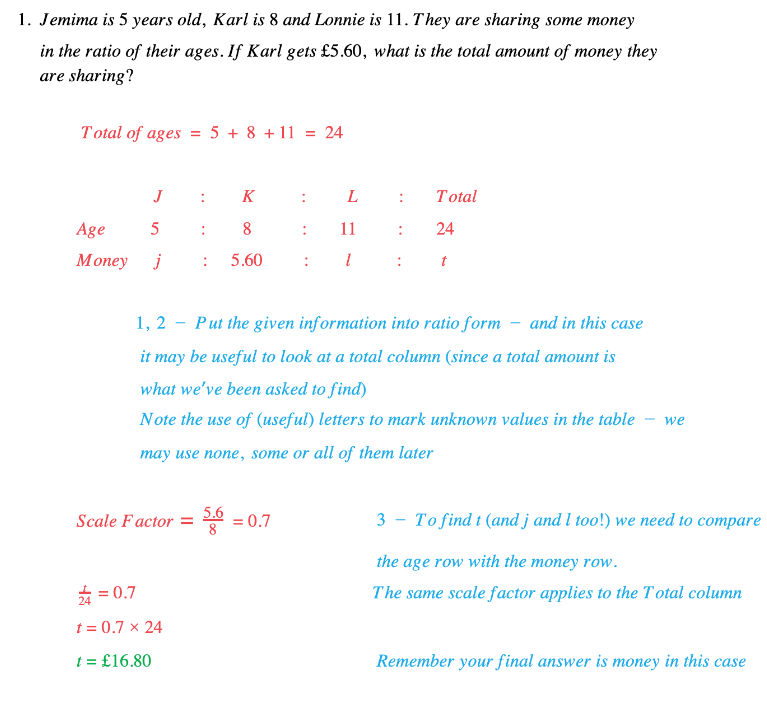# Edexcel IGCSE Maths 复习笔记 1.11.1 Ratios

Edexcel IGCSE Maths 复习笔记 1.11.1 Ratios

#### What is a ratio?

• ratio is a way of comparing one part of a whole to another
• ratio can also be expressed as a fraction (of the whole)
• We often use a ratio (instead of a fraction) when we are trying to show how things are shared out or in any situation where we might use scale factors

#### How to work with ratios

1. Put what you know in RATIO form (use more than one line if necessary)
2. Add “extra bits” (eg Total, Difference, Sum) if you think they might be useful
3. Use SCALE FACTORS to complete lines